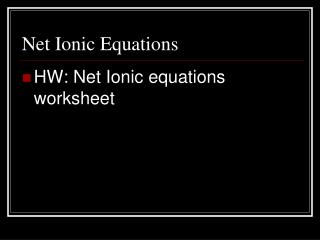DownloadDownload PresentationNet Ionic Equations

# Net Ionic Equations

Télécharger la présentation## Net Ionic Equations

- - - - - - - - - - - - - - - - - - - - - - - - - - - E N D - - - - - - - - - - - - - - - - - - - - - - - - - - -
##### Presentation Transcript

1. Net Ionic Equations • HW: Net Ionic equations worksheet

2. Net Ionic Equations • Most ionic compounds dissociate completely into cations and anions when they dissolve in water. • Written for double and single replacement reactions

3. Net Ionic Equations • Write the complete ionic equation (includes all dissolved ionic compounds as their free ions.) Cl2 (g) + 2NaBr (aq) → Br2(l) + 2NaCl (aq)

4. Complete Ionic Equations (step 1) Cl2 (g) + 2NaBr (aq) → Br2(l) + 2NaCl (aq) Cl2(g) + 2Na+(aq) + 2Br-(aq)→ Br2(l) + 2Na+(aq) + 2Cl-(aq)

5. Spectator ions (step 2) • Spectator ion: not directly involved in a reaction. Appears on both sides of the equation. • In the previous problem, 2Na+(aq) is the spectator ion.

6. Net ionic equation (step 3) • Indicates only the particles that actually take part in the reaction. Cl2(g) + 2Br-(aq)→ Br2(l) + 2Cl-(aq) • Make sure that the charges balance on both sides of the equation

7. Net Ionic Equation (Example) • Solutions of Lead(II) acetate and cupric sulfate are mixed. • Write the balanced equation • Write the complete ionic equation • Identify the spectator ions • Write the net ionic equation

8. Balanced Equation Pb(C2H3O2)2(aq) + CuSO4(aq) → PbSO4(s) + Cu(C2H3O2)2(aq)

9. Complete Ionic Equation Pb2+(aq) + 2C2H3O2-(aq) + Cu2+(aq) + SO42-(aq)→ PbSO4(s) + Cu2+(aq) + 2 C2H3O2-(aq) Note: PbSO4 does not dissolve in water!

10. Identify Spectator Ions • 2C2H3O2-(aq) • Cu2+(aq) Appear on both sides of the equation

11. Net Ionic equation • Eliminate spectator ions Pb2+(aq) + SO42-(aq) → PbSO4(s) Note: zero net charge on reactant side; zero net charge on product side